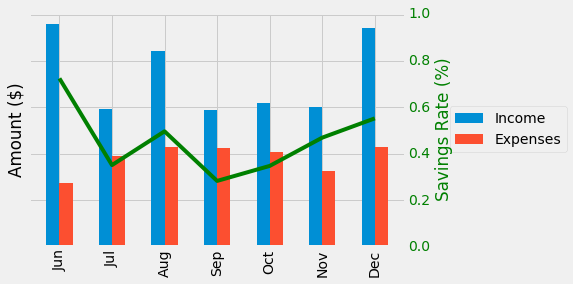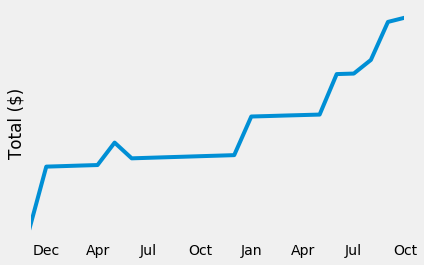# Using python to plot ledger transactions

ledger is a very capable piece of software for double-entry accounting. However, it only provides one out-of-the-box option for visualizing transactions: gnuplot. Rather than learn a non-FOSS tool (gnuplot is not really GNU), I set out to get ledger transactions into pandas. `ledger` includes python bindings which worked for 95% of my plotting needs, but some missing journal properties were ultimately a showstopper. Fortunately, the `gnuplot` flags (-J and -j) produce output almost ready to be parsed by `pandas`.

#### Using python-ledger

The python bindings are pleasant to use, but require some work to get into `pandas`.

``````# Necessary imports
import matplotlib.pyplot as plt
import ledger
import pandas as pd

``````

Queries are performed to find transactions after the `journal` is read.

``````# Find all Income transactions
posts = journal.query("Income")
``````

However, `posts` is not easily digestible by pandas. It is possible to build a parseable list by iterating through each transaction:

``````income = []
for post in journal.query("Income"):
trans = {'Income':post.amount,'Datetime':post.date}
income.append(trans)
incomeDF = pd.DataFrame(income)
``````

The current `incomeDF` has a standard index (1,2,...X) and the `Datetime` column.

Datetime Income
0 2016-11-04 \$-115.23
1 2016-12-01 \$-144.04
... ... ...
543 2017-04-24 \$-100.00

The standard index can be replaced with `Datetime` allowing Grouper methods to be used.

``````incomeDF = incomeDF.set_index(pd.DatetimeIndex(incomeDF['Datetime']))
``````
Income
Datetime
2016-11-04 \$-115.23
2016-12-01 \$-144.04
... ...
2017-04-24 \$-100.00

The `Income` data is a string and cannot be plotted with pandas. The '\$' can be removed and the new string cast as a float.

``````incomeDf['Income'] = incomeDF['Income'].replace('[\\$]','',regex=True).astype(float)
``````

With the transactions in a dataframe anything is possible.

Since the index is `Datetime` the transactions can be grouped by month and summed.

``````monthly = df.groupby(pd.TimeGrouper(freq='M'))
monthly = monthly.sum().ix['2016-06-01':'2016-12-31'].fillna(0).abs()
``````

Creating a dataframe for "Expenses" and combining it with `incomeDF`
(`pd.concat([incomeDF,expensesDF],join='outer')`) allows a plot comparing Income and Expenses to be made.

``````plt.style.use('fivethirtyeight')
ax = monthly.loc[:,['Income','Expenses']].plot.bar()
ax.set_xticklabels([dt.strftime('%b') for dt in monthly.index.to_pydatetime()])
plt.xlabel('')
plt.title('Income and Expenses Comparison')
rate = 1-(monthly['Expenses'])/monthly['Income']
ax2 = ax.twinx()
ax2.plot(rate.values,'g')
#ax.grid(False)
ax2.grid(False)
ax2.set_ylim([0,1])
ax.legend(loc='center left',bbox_to_anchor=(1.1,0.5))
ax.set_ylabel('Amount (\$)')
ax2.set_ylabel('Savings Rate (%)', color='g')
ax2.tick_params('y',colors='g')
``````#### Using gnuplot output

The `-j` and `-J` flags cause ledger to produce `gnuplot` friendly output. Fortunately, this output is straightforward: a date column and a transaction column. The `-J` provides a running total while `-j` produces each individual transaction amount.

``````...
2017-01-13 144.04
2017-01-13 1438.31
2017-01-13 450
...
``````

Calling the `ledger` binary from python is accomplished using the subprocess module.

``````import subprocess as sp
import io
import pandas as pd

# Run ledger using subprocess
ledger_file = '/data/ledger/finances.ldg'
result = sp.run('ledger -f {} -JMRV reg Assets'.format(ledger_file),shell=True,stdout=sp.PIPE)
output = result.stdout.decode('ascii')
``````

The results from `sp.run` are binary encoded and must be decoded to ASCII for python string operations to work.

Pandas' `read_csv` expects to read a file. Fortunately, `io` provides a way for an in-memory string to be treated as a file with `StringIO`.

``````# 'Fake' csv
f = io.StringIO(output)

# Create Dataframe
``````df.loc['2007-12-01':'2009-10-01'].plot()Was this information useful or thought provoking? Do you appreciate a webpage free of analytics or ads? Say thanks and help keep this site online by using my Amazon Affilliate URL . I'll receive a small percentage of purchases made within 24 hours of clicking. Or, feel free to donate BTC (`1DNwgPQMfoWZqnH78yt6cu4WukJa3h8P1f`) or ETH (`0xf3c4a78c24D34E111f272Ac2AC72b1f01ba52DF3`).